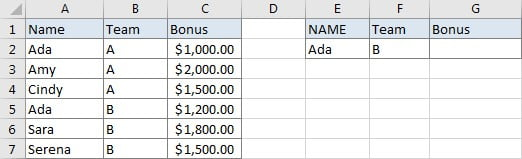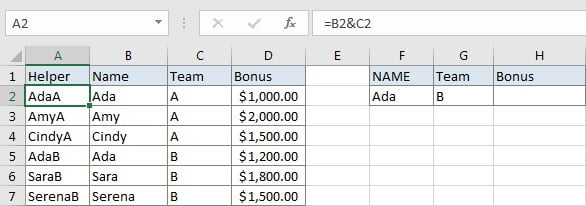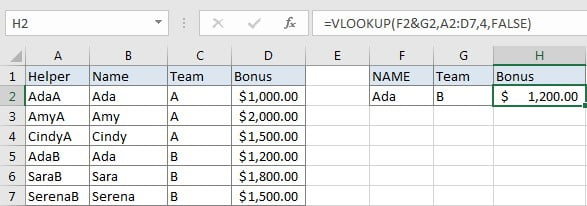# VLOOKUP with Multiple Lookup Values

VLOOKUP is one of the key functions among all lookup & reference functions in Excel. It can scan and retrieve data from a static or dynamic table based on your lookup value. It can perform approximate match or exact match by setting lookup matching modes.

Today, we will show you the way to use VLOOKUP to retrieve data depend on two lookup values.

## EXAMPLEIn this instance, we want to know the bonus for the entered employee “Ada” in E2. We can see that there are two “Ada” listed in the first column of the left table, they belong to different teams. So, to calculate bonus exactly, we add “Team” as another condition. Now, there are two items “Name” and “Team” to determine which bonus should be returned, it is equivalent to there are two lookup values in the table. As VLOOKUP function cannot handle two lookup values, so we need to add a helper column to help us concentrate the two values “Name” and “Team” into one, then we can through searching “AdaB” from this helper column to get proper bonus.

As VLOOKUP scans lookup value in the first column of lookup table, so the helper column must be the first column. We use “&” to concentrate name and team and save them in helper column.## ANALYSIS

Construct a formula with VLOOKUP function containing two lookup values, we can refer to below points:

a. Concentrate the two used columns “Name” & “Team”, and create a helper column to save concentrated values. This helper column must be inserted to the left to column “Name”. Lookup table contains the helper column.

b.Set “lookup value1&lookup value2” as your lookup value.

c. VLOOKUP function performs an exact match lookup.

Now we can set up VLOOKUP based on the new lookup value and lookup table now.

## FORMULA

Input formula =VLOOKUP(F2&G2,A2:D7,4,FALSE) into E2, press ENTER, verify that “1200” is returned properly.## FUNCTION INTRODUCTION

x
How to Select Every Other Row in Excel

a. VLOOKUP function looks up the input value from the first column of the given table, and returns the value we want from a particular position (row number depends on the row of lookup value, column number depends on the input argument col_index_num).

Syntax:

=VLOOKUP(lookup_value, table_array, col_index_num,[range_lookup])

For argument “range_lookup”, there are two matching modes “True” and “False”.

• The default mode is “True”, so if this optional argument is omitted, this value is set to “True” automatically.
• If we set “range_lookup” to “True”, VLOOKUP function performs an approximate match.
• If we set “range_lookup” to “False”, VLOOKUP function looks up data restrictively by performing an exact match. If look up value is not found in table, formula returns error “#N/A”.

## EXPLANATION

`=VLOOKUP(F2&G2,A2:D7,4,FALSE)`

lookup_value is F2&G2

table_array is A2:D7

col_index_num is 4. Because bonuses are saved in the fourth column of this table.

range_lookup is set to FALSE. VLOOKUP function performs an EXACT match.

a. In this formula, the look up value is “F2&G2” (“AdaB”), combination of the two lookup values. VLOOKUP scans this value from the first column of lookup table A2:D7. It continues scanning until it meets the same string. In this case, VLOOKUP function will stop scanning when meeting “AdaB” in cell A5.

b. The lookup table is range A2:D7. The helper column is listed in the first column.

c. As “Bonus” column is the fourth column in lookup table, so we enter 4 as col_index_num. VLOOKUP function retrieves data in this column after confirming the position of lookup value.

d. We set range_lookup mode to “FALSE”, so VLOOKUP function performs an exact match lookup and this operation can make sure that VLOOKUP returns correct bonus value. If lookup value cannot be found in the given table, an error #N/A will be returned.

### Related Functions

• Excel INDEX function
The Excel INDEX function returns a value from a table based on the index (row number and column number)The INDEX function is a build-in function in Microsoft Excel and it is categorized as a Lookup and Reference Function.The syntax of the INDEX function is as below:= INDEX (array, row_num,[column_num])…
• Excel VLOOKUP function
The Excel VLOOKUP function lookup a value in the first column of the table and return the value in the same row based on index_num position.The syntax of the VLOOKUP function is as below:= VLOOKUP (lookup_value, table_array, column_index_num,[range_lookup])….

Related Posts

VBA Macro For VLOOKUP From Another Sheet

In the previous post, you should know that how to fix or remove the #N/A error when using VLOOKUP formula to lookup value from another sheet. And this post will show you how to use VBA code to vlookup data ...

VLOOKUP From Another Sheet Not Working

In the previous post, you should know that how to fix or remove the #N/A error when using VLOOKUP formula to lookup value from another sheet. And this post will show you reasons why your VLOOKUP formula is not working ...

Fix #N/A Error For VLOOKUP From Another Sheet

This post will show you how to fix the #N/A error why it occurs when you extract values from another sheet using VLOOKUP function in Excel 2016,2013,2010 or other Excel versions. How can you correct a #N/A error in VLOOKUP ...

Get Employee Information by VLOOKUP

VLOOKUP is one of the key functions among all lookup & reference functions in Excel. Today, in this article, we will show you the way to apply VLOOKUP to retrieve employee information. I hope this article will help you in ...

VLOOKUP with Two Lookup Tables

VLOOKUP is one of the key functions among all lookup & reference functions in Excel. Today we will show you the application of VLOOKUP function when there are two lookup tables. EXAMPLE Table1 and table2 record the rates of Y2020 ...

VLOOKUP Data by Date

VLOOKUP is one of the key functions among all lookup & reference functions in Excel. It can scan and retrieve data from a static or dynamic table based on your lookup value. It can perform approximate match or exact match ...

VLOOKUP – Retrieve Data from Another Workbook

VLOOKUP is one of the key functions among all lookup & reference functions in Excel. It can scan and retrieve data from a static or dynamic table based on your lookup value. It can perform approximate match or exact match ...

VLOOKUP – Retrieve Data from Another Worksheet

VLOOKUP is one of the key functions among all lookup & reference functions in Excel. It can scan and retrieve data from a static or dynamic table based on your lookup value. It can perform approximate match or exact match ...

Case Sensitive Lookup with SUMPRODUCT and EXACT

Today, we will show you how to use SUMPRODUCT and EXACT to perform a case sensitive exact match. In this article, we provide a simple example to calculate bonus for employees whose names are case-sensitive. If you meet similar scenarios ...

Basic Grade Calculation by VLOOKUP Function – Approximate Match

In Excel, except combination INDEX+MATCH, we can also apply other functions to search data, for example VLOOKUP function. Like MATCH function, VLOOKUP function is one of Excel lookup & reference functions that can perform approximate match or exact match by ...

Sidebar